## Thursday, April 13, 2017

### Computing a phylogenetic 'traitgram' in phytools when one but not all internal node states are known

The phytools function `phenogram` can take as input the trait values for the tips of the tree (in which case internal nodes will be estimated using ML) or the values for all tips & internal nodes, such as we might have from simulation.

In the former & latter cases, respectively, the names of our input vector should match the tip labels or the tip labels & internal node indices of our `"phylo"` or `"simmap"` class object.

For instance, in the following tree & data `x` contains all the tip & node states, whereas `y` contains just the terminal states:

``````library(phytools)
tree
``````
``````##
## Phylogenetic tree with 26 tips and 25 internal nodes.
##
## Tip labels:
##  A, B, C, D, E, F, ...
##
## Rooted; includes branch lengths.
``````
``````x
``````
``````##            A            B            C            D            E
##  0.829142741  1.049240326  1.676064680  1.103328482  2.741820966
##            F            G            H            I            J
##  3.218365580  2.325949799  1.508513542  2.873934462 -0.119931801
##            K            L            M            N            O
##  0.293037949  2.233414058  0.807865584  1.541024146  1.195199822
##            P            Q            R            S            T
## -0.417434223  0.006972801  0.193993966  1.615198461  0.775281744
##            U            V            W            X            Y
##  1.526512067  2.924548100  2.859262928  2.267024775  2.416482578
##            Z           27           28           29           30
## -0.863556075  0.000000000  1.428034695  1.880731653  1.043718793
##           31           32           33           34           35
##  1.939163010  1.625363493  2.166216386  2.311081321  3.103867929
##           36           37           38           39           40
##  1.598404653  2.353648451  1.438533276  0.240418088  0.711084655
##           41           42           43           44           45
##  1.243855279  1.342363084  0.334593068  0.526738468  0.029314698
##           46           47           48           49           50
##  0.708468995  1.980958676  2.069855863  2.292636798  2.962467736
##           51
##  1.744952751
``````
``````y
``````
``````##            A            B            C            D            E
##  0.829142741  1.049240326  1.676064680  1.103328482  2.741820966
##            F            G            H            I            J
##  3.218365580  2.325949799  1.508513542  2.873934462 -0.119931801
##            K            L            M            N            O
##  0.293037949  2.233414058  0.807865584  1.541024146  1.195199822
##            P            Q            R            S            T
## -0.417434223  0.006972801  0.193993966  1.615198461  0.775281744
##            U            V            W            X            Y
##  1.526512067  2.924548100  2.859262928  2.267024775  2.416482578
##            Z
## -0.863556075
``````
``````phenogram(tree,x)
``````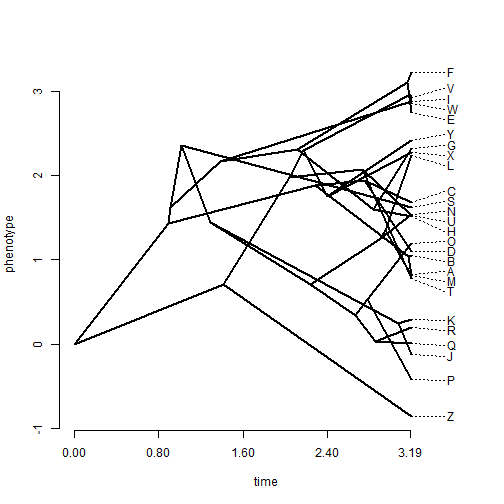``````phenogram(tree,y)
``````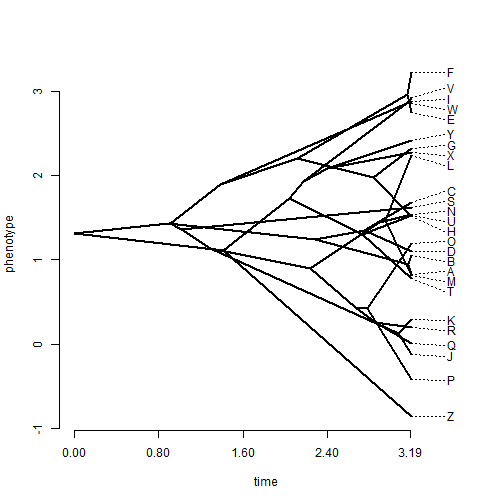If the second of the two plots seems neater - it is simply because the ML estimated states tend to be intermediate between the two descendent tip or node values, though in reality the true states will not always be so neatly positioned. The following shows a more realistic portrait of our confidence in ancestral states estimated using `phenogram` (called a `phenogram95` in phytools), but with the true states overlain (since we happen to know them in this case):

``````fancyTree(tree,type="phenogram95",x=y)
``````
``````## Computing density traitgram...
``````
``````phenogram(tree,x,add=TRUE,ftype="off")
``````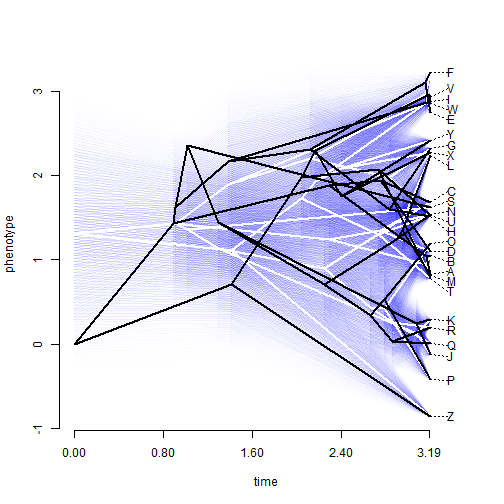Sometimes, however, we might want to provide `phenogram` with not all internal node states - but with the state at one or a small number of particular nodes. Unfortunately, this gets a little complicated. For instance, if we just try to supply these values to `phenogram`, labeled as above, it will simply not work. E.g.,:

``````z<-x[1:27]
z
``````
``````##            A            B            C            D            E
##  0.829142741  1.049240326  1.676064680  1.103328482  2.741820966
##            F            G            H            I            J
##  3.218365580  2.325949799  1.508513542  2.873934462 -0.119931801
##            K            L            M            N            O
##  0.293037949  2.233414058  0.807865584  1.541024146  1.195199822
##            P            Q            R            S            T
## -0.417434223  0.006972801  0.193993966  1.615198461  0.775281744
##            U            V            W            X            Y
##  1.526512067  2.924548100  2.859262928  2.267024775  2.416482578
##            Z           27
## -0.863556075  0.000000000
``````
``````phenogram(tree,z)
``````
``````## Error in ace(x, a, method = "pic"): length of phenotypic and of phylogenetic data do not match.
``````

We can, however, compute the ML internal node states (taking our known values into account) using an external function & then supply these quantities to `phenogram`. This could look something like the following:

``````a<-x[c("27","37","40")] ## root node plus nodes 37 & 40
a
``````
``````##        27        37        40
## 0.0000000 2.3536485 0.7110847
``````
``````obj<-fastAnc(tree,y,anc.states=a)
obj
``````
``````## Ancestral character estimates using fastAnc:
##       27       28       29       30       31       32       33       34
## 0.000000 2.052928 1.379978 0.946563 1.386517 2.100724 2.294888 2.361165
##       35       36       37       38       39       40       41       42
## 2.968568 2.006992 2.353648 1.787318 0.140612 0.711085 1.411087 1.442027
##       43       44       45       46       47       48       49       50
## 0.385406 0.386695 0.233705 0.713551 1.589630 1.260287 1.823774 2.888929
##       51
## 2.016556
``````
``````phenogram(tree,c(y,obj))
``````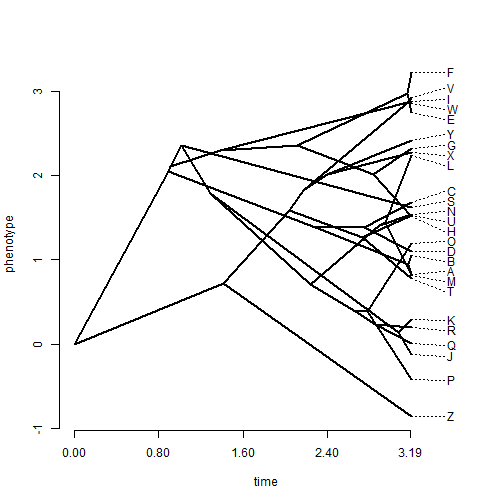Now let's overlay this reconstruction on the known true values:

``````phenogram(tree,x,col=make.transparent("blue",0.4))
par(fg="transparent")
nodelabels(pie=matrix(1,nrow=tree\$Nnode),cex=0.25,
piecol="blue")
ftype="off")
nodelabels(pie=matrix(1,nrow=tree\$Nnode),cex=0.25,
piecol="red")
``````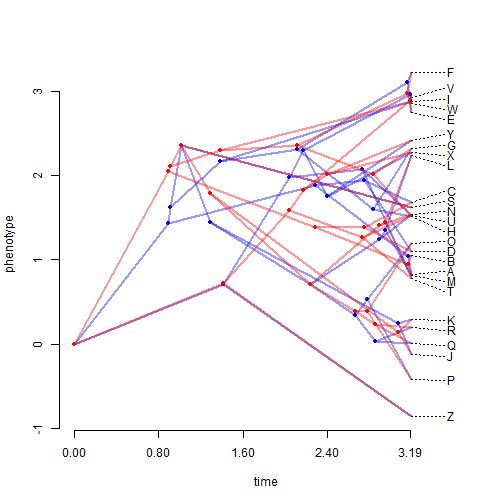``````par(fg="black")
``````

That's it.